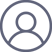刘全慧2020-9-24 10:00

《热力学与统计物理》第一课，谢同学就热力学第零定律提出一个问题。${{g}_{A}}({{p}_{A}},{{V}_{A}})={{g}_{B}}({{p}_{B}},{{V}_{B}})$            (1)

${{g}_{A}}({{p}_{A}},{{V}_{A}})={{g}_{C}}({{p}_{C}},{{V}_{C}})$            (2)

${{g}_{A}}({{p}_{A}},{{V}_{A}})={{g}_{B}}({{p}_{B}},{{V}_{B}}) ={{g}_{C}}({{p}_{C}},{{V}_{C}})=T$         (3)